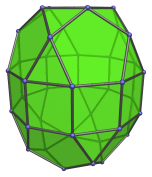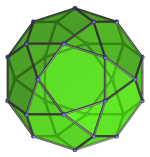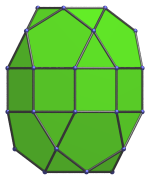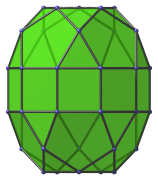# The Elongated Pentagonal Gyrobirotunda

The elongated pentagonal gyrobirotunda is the 43rd Johnson solid (J43). It has 40 vertices, 80 edges, and 42 faces (20 equilateral triangles, 10 squares, 12 pentagons).The elongated pentagonal gyrobirotunda can be constructed by attaching two pentagonal rotundae to a decagonal prism, or equivalently, inserting a decagonal prism between the two halves of an icosidodecahedron. The gyro- in the name refers to how the top and bottom pentagons are rotated with respect to each other. If they are aligned to each other instead, the elongated pentagonal orthobirotunda (J42) is produced instead.

## Projections

Here are some views of the elongated pentagonal gyrobirotunda from various angles:

Projection DescriptionTop view.Front view.Side view.

## Coordinates

The Cartesian coordinates of the elongated pentagonal gyrobirotunda with edge length 2 are:

• (−√((10+2√5)/5), 0, 1+√((20+8√5)/5))
• (−√((5−√5)/10), ±φ, 1+√((20+8√5)/5))
• ( √((5+2√5)/5), ±1, 1+√((20+8√5)/5))
• ( √((20+8√5)/5), 0, 1+√((10+2√5)/5))
• (−√((25+11√5)/10), ±φ, 1+√((10+2√5)/5))
• ( √((5+√5)/10), ±φ2, 1+√((10+2√5)/5))
• (±√(3+4φ), ±1, ±1)
• (±√(2+φ), ±φ2, ±1)
• (0, ±2φ, ±1)
• (−√((20+8√5)/5), 0, −(1+√((10+2√5)/5)))
• ( √((25+11√5)/10), ±φ, −(1+√((10+2√5)/5)))
• (−√((5+√5)/10), ±φ2, −(1+√((10+2√5)/5)))
• ( √((10+2√5)/5), 0, −(1+√((20+8√5)/5)))
• ( √((5−√5)/10), ±φ, −(1+√((20+8√5)/5)))
• (−√((5+2√5)/5), ±1, −(1+√((20+8√5)/5)))

where φ=(1+√5)/2 is the Golden Ratio.

Last updated 03 Sep 2018.